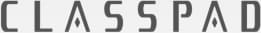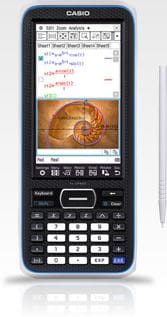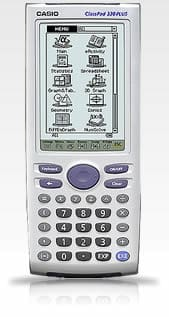Code CP400
SRP Price\$279.00 inc. GST
Description

### Overview - WACE Approved

The ClassPad series continues to evolve as the next generation machine is optimised for use in the classroom. The new fx-CP400 is the first ClassPad model to boast a 4.8 inch colour display. The fx-CP400 offers intuitive stylus and finger touch operation. It also supports horizontal screen view. Simply switch the display between vertical and horizontal screen views by a touch on the icon panel.   Main features include:

• 4.8" colour display
• Finger or stylus touch operation
• Horizontal screen view
• Simple, intuitive user interface
Specificationsfx-CP400 OS 2.00ClassPad 330 PLUS OS 3.10 Hardware LCD Color more than 65,000 colors monochrome Size 4.8 inch 3.6inch Dot Matrix 320*528 160*240 Touch Panel X X Dimension 206.0 x 89.0 x 21.1 mm 189.5 x 84 x 21 mm Weight 320g 280g USB X X 3Pin X X Horizontal View X - Battery Type AAA*4 Alkaline/Ni-MH AAA*4 Alkaline/Ni-MH Battery Duration 100h 140h User Memory Flash Memory 24MB 6MB Flash Memory2 for eActivity 5.5MB 5.5MB RAM 500kB 500kB Usability Multi-Window X X Drag&Drop Operation X X Pinch-in / Pinch-out(Graph&Table, Conics) X - Slider X - Functions Calculation CAS X X Laplace/Fourie/Delta/Gamma X X Complex X X List X X Matrix X X Vector X X Vector Equation X - Base-n X X User Defined function X X Main/Calculator Application X X Interactive menu X X Statistics X X 1-Var/2-Var X X Regression X X Test/Interval/Probability X X Stat Graph X X Spreadsheet X X 1-Var/2-Var X X Regression X X Test/Interval/Probability X X Box Plot/Histogram X X Scatter/XY Line X X Bar Chart/Pie Chart X X Probability Graph X X Graph by Numeric Data X X Graph/Sheet Color Settings X X Color Link X - Cell Operation (If/Sum...\$A) X X Import / Export csv X - Graph&Table X X y= graph X X r= graph X X Parameter graph X X X= graph X X Inequality Graph X X Modify X X Trace/Zoom X X Root/Intersect/MinMax/Integral X X Tangent X X Grid/Axes X - Background Picture X - Table X X Summary Table X X 3D Graph X X Conics X X Sequence X X DiffEq y'= X X x'=/y'= X X y1'=/y2'= X X Geometry X X Object Drawing X X Object Handling X X Construction/Transformation X X Graph X X Measurement X X Animation/Slider X X Object Color Settings X - PicturePlot X - Interactive DiffCalc X - NumSolve X X Financial X X Program X X Documantation Tool eActivity eActivity Communication Handheld to Handheld X X Handheld to PC / PC to Handheld USB Mass Storage USB Mass Storage Screen Capture Screen Receiver Software Screen Receiver Software Direct Projector Connection X * X * Sensor Connection E-CON2 E-CON2 Physium X(Add-in) - Settings KeyboardSetting Qwerty/Azerty/Qwertz/ABC X X Imaginary Unit X X Custom Key Settings X X Language English/German/French/Spanish/Finnish X X
Warranty

Manufacture warranty 12 months

Abacus extend to 2 year warranty